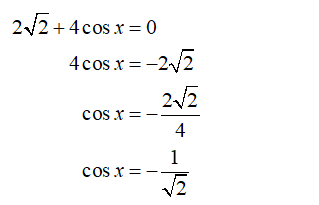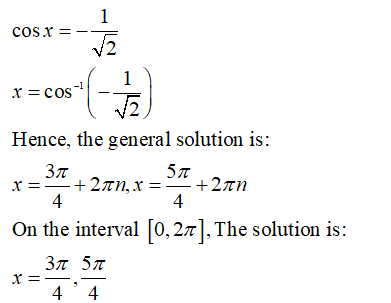Question
4 views

Solve the folowing equation on [0,2pi)

2sqrt2 +4cosx=0

check_circle

Step 1

In the given equation, the cosine function is the trigonometric function:

Rewrite the given equation:Step 2

The cosine function is negative in the second and third quadrant:...

### Want to see the full answer?

See Solution

#### Want to see this answer and more?

Solutions are written by subject experts who are available 24/7. Questions are typically answered within 1 hour.*

See Solution
*Response times may vary by subject and question.
Tagged in